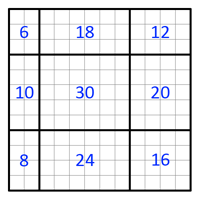Area Magic Squares
Area magic squares are an idea of William Walkington.

 Geometrical Interpretation of matricesArea Matrix Definition (PDF) An Area Matrix (A,M) is a combination of a positive matrix A and a set M of continuous graphs. An Area Magic Square (AMS) is an area matrix (A,M) where the matrix A is a magic square. Less mathematical definitions (PDF)

 Linear Area Magic Squares of order 3 Third-Order Linear Area Magic Squares - Algorithm (PDF)
The following AMS were calculated with this algorithm. The graphics were made in high resolution by William Walkington.
Shown is each of the two solutions for magic 3x3 squares with consecutive numbers.
Click on a small picture to see it in a new frame, then click again to enlarge it.
 2017-01-06: First Third-Order Linear Area Magic Square (PDF) Theory of area magic squares (PDF) Third-Order Integer Area Square (PDF)Curved Area Magic Square (PDF)

 Linear Area Magic Squares of order 4 2017-01-14: First Order-4 Linear Area Magic Square Article: Semi-orthogonal linear area magic squares of order 4 - part 1 (PDF)
1 What is a linear area magic square?
2 When were linear area magic squares first found?
3 How to present the number matrix?
4 What are the properties of the number matrix?
5 How many numbers or parameters are sufficient?
6 How can we describe all numbers by parameters?
7 How to avoid duplicates?
8 How to construct all L-AMS for a certain magic sum S?
9 What can be calculated with a computer?         How to draw a semi-orthogonal linear area magic square of order 4?
How can we determine the distances?
Several examples.
Examples (animated GIF)
 Other Area Magic Squares of order 4

 Area Magic Squares of order 5 Semi-orthogonal linear area magic squares of order 5 do not exist. (Equal areas would occur) If linear area magic squares of order 5 exist, they would consist of large entries.

Many other magic area squares (also orders > 4) were found by William Walkington and Hans-Bernhard Meyer.
 Links Hans-Bernhard Meyer: Observations on 4x4 Area Magic Squares with vertical Lines William Walkington: Area Magic Squares and Tori of order 3 Area Magic Squares of order 6 Area Magic Squares of order 4 Carlos Rivera: Area Magic Squares with prime numbers (solutions by Jan van Delden)

 Home: Notes on Magic Squares Walter Trump, Nürnberg, Germany, (c) 2017-01-28, last update 2018-06-19